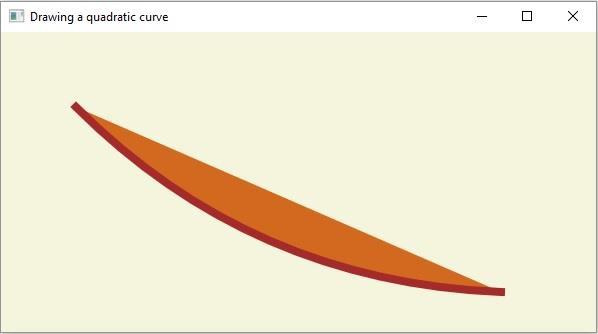# How to create a QuadCurve using JavaFX?

A quadratic curve is a Bezier parametric curve in the XY plane of degree 2.

In JavaFX, a circle is represented by the javafx.scene.shape.QuadCurve class. It is similar to the CubicCurve but instead of 2, it is drawn using one control point.

This class contains 6 properties they are −

• startX − This property represents the x coordinate of the starting point of the curve. You can set the value to this property using the setStartX() method.

• startY − This property represents the y coordinate of the starting point of the curve. You can set the value to this property using the setStartY() method.

• controlX − This property represents the x coordinate of the control point of the curve. You can set the value to this property using the setControlX() method.

• controlY − This property represents the y coordinate of the control point of the curve. You can set the value to this property using the setControlY() method.

• endX − This property represents the x coordinate of the endpoint of the curve. You can set the value to this property using the setEndX() method.

• endY − This property represents the y coordinate of the endpoint of the curve. You can set the value to this property using the setEndY() method.

To create a circle you need to −

• Instantiate this class.

• Set the required properties using the setter methods or, bypassing them as arguments to the constructor.

• Add the created node (shape) to the Group object.

## Example

import javafx.application.Application;
import javafx.scene.Group;
import javafx.scene.Scene;
import javafx.scene.paint.Color;
import javafx.stage.Stage;
public class DrawingQuadCurve extends Application {
@Override
public void start(Stage stage) {
//Setting properties to cubic curve
qudraticCurve.setStartX(75.0f);
qudraticCurve.setStartY(75.0f);
qudraticCurve.setControlX(250.0f);
qudraticCurve.setControlY(250.0f);
qudraticCurve.setEndX(500.0f);
qudraticCurve.setEndY(260.0f);
//Setting other properties
qudraticCurve.setFill(Color.CHOCOLATE);
qudraticCurve.setStrokeWidth(8.0);
qudraticCurve.setStroke(Color.BROWN);
//Setting the scene object
Group root = new Group(qudraticCurve);
Scene scene = new Scene(root, 595, 300);
stage.setScene(scene);
stage.show();
}
public static void main(String args[]){
launch(args);
}
}

## Output